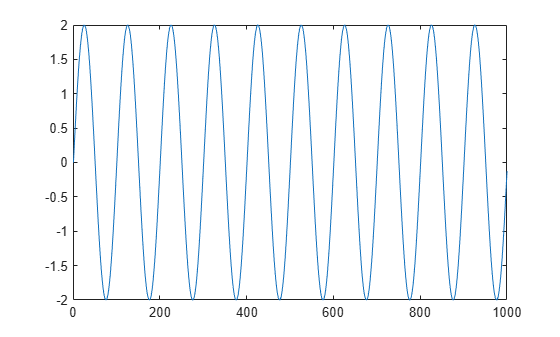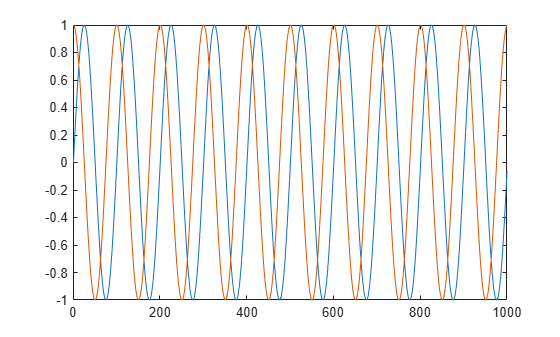Documentation

# dsp.SineWave

Generate discrete sine wave

## Description

The `dsp.SineWave` System object™ generates a real or complex, multichannel sinusoidal signal with independent amplitude, frequency, and phase in each output channel.

For both real and complex sinusoids, the Amplitude, Frequency, and PhaseOffset properties can be scalars or length-N vectors, where N is the number of channels in the output. When you specify at least one of these properties as a length-N vector, scalar values specified for the other properties are applied to each of the N channels.

To generate a discrete-time sinusoidal signal:

1. Create the `dsp.SineWave` object and set its properties.

2. Call the object with arguments, as if it were a function.

To learn more about how System objects work, see What Are System Objects? (MATLAB).

## Creation

### Syntax

``sine = dsp.SineWave``
``sine = dsp.SineWave(Name,Value)``
``sine = dsp.SineWave(amp,freq,phase,Name,Value)``

### Description

````sine = dsp.SineWave` creates a sine wave object that generates a real-valued sinusoid with an amplitude of 1, a frequency of 100 Hz, and a phase offset of 0. By default, the sine wave object generates only one sample.```
````sine = dsp.SineWave(Name,Value)` creates a sine wave object with each specified property set to the specified value. Enclose each property name in single quotes. Example: sine = dsp.SineWave('Amplitude',2);```

example

````sine = dsp.SineWave(amp,freq,phase,Name,Value)` creates a sine wave object with the Amplitude property set to `amp`, Frequency property set to `freq`, PhaseOffset property set to `phase`, and anyother specified properties set to the specified values.```

## Properties

expand all

Unless otherwise indicated, properties are nontunable, which means you cannot change their values after calling the object. Objects lock when you call them, and the `release` function unlocks them.

If a property is tunable, you can change its value at any time.

For more information on changing property values, see System Design in MATLAB Using System Objects (MATLAB).

Amplitude of the sine wave, specified as one of the following:

• scalar –– A scalar applies to all channels.

• vector –– A length-N vector contains the amplitudes of the sine waves in each of the N output channels. The vector length must be the same as that specified for the Frequency and PhaseOffset properties.

Tunable: Yes

#### Dependencies

This property is tunable only when you set Method to either ```'Trigonometric function'``` or `'Differential'`.

Data Types: `single` | `double` | `int8` | `int16` | `int32` | `int64` | `uint8` | `uint16` | `uint32` | `uint64`

Frequency of the sine wave in Hz, specified as one of the following:

• scalar –– A scalar applies to all channels.

• vector –– A length-N vector contains the frequencies of the sine waves in each of the N output channels. The vector length must be the same as that specified for the Amplitude and PhaseOffset properties.

Data Types: `single` | `double` | `int8` | `int16` | `int32` | `int64` | `uint8` | `uint16` | `uint32` | `uint64`

Phase offset of the sine wave in radians, specified as one of the following:

• scalar –– A scalar applies to all channels.

• vector –– A length-N vector contains the phase offsets of the sine waves in each of the N output channels. The vector length must be the same as that specified for the Amplitude and Frequency properties.

Data Types: `single` | `double` | `int8` | `int16` | `int32` | `int64` | `uint8` | `uint16` | `uint32` | `uint64`

Flag that indicates whether the waveform is real or complex, specified as either:

• `false` –– The waveform output is real.

• `true` –– The waveform output is complex.

Method used to generate sinusoids, specified as one of the following:

• `'Trigonometric function'` –– The object computes the sinusoid by sampling the continuous-time function.

• `'Table lookup'` –– The object precomputes the unique samples of every output sinusoid at the start of the simulation, and recalls the samples from memory as needed.

• `'Differential'` –– The object uses an incremental algorithm. This algorithm computes the output samples based on the output values computed at the previous sample time and precomputed update terms.

Optimize table of sine values for speed or memory, specified as either:

• `'Speed'` –– The table contains k elements, where k is the number of input samples in one full period of the sine wave. The period of each sinusoid must be an integer multiple of 1/Fs, where Fs is the value of the SampleRate property value. That is, each element of the Frequency property must be of the form Fs/m, where m is an integer greater than `1`.

• `'Memory'` –– The table contains k/4 elements.

#### Dependencies

This property applies only when you set the `Method` property to `'Table lookup'`.

Sample rate of output signal in Hz, specified as a positive scalar.

Example: `44100`

Example: `22050`

Number of consecutive samples from each sinusoid to buffer into the output frame, specified as a positive integer.

Example: `1000`

Example: `5000`

Data Types: `single` | `double` | `int8` | `int16` | `int32` | `int64` | `uint8` | `uint16` | `uint32` | `uint64`

Data type of the sine wave output, specified as `'double'`, `'single'`, or `'Custom'`.

#### Fixed-Point Properties

Output word and fraction lengths, specified as an autosigned numeric type with a word length of 16.

Example: numerictype([],32,30)

Example: numerictype([],16,15)

#### Dependencies

This property applies only when you set the Method property to ```'Table lookup'``` and the OutputDataType property to `'Custom'`.

## Usage

For versions earlier than R2016b, use the `step` function to run the System object algorithm. The arguments to `step` are the object you created, followed by the arguments shown in this section.

For example, `y = step(obj,x)` and `y = obj(x)` perform equivalent operations.

### Syntax

``sineOut = sine()``

### Description

example

````sineOut = sine()` creates the sine wave output, `sineOut`.```

### Output Arguments

expand all

Sine wave output, returned as a vector or matrix. The SamplesPerFrame property determines the number of rows in the output matrix. If the Frequency or the PhaseOffset property is a vector, the length of the vector determines the number of columns (channels) in the output matrix. If the `Frequency` or the `PhaseOffset` properties is a scalar, then the number of channels in the output matrix is 1.

The OutputDataType property sets the data type of the output.

Data Types: `single` | `double` | `fi`

## Object Functions

To use an object function, specify the System object as the first input argument. For example, to release system resources of a System object named `obj`, use this syntax:

`release(obj)`

expand all

 `step` Run System object algorithm `release` Release resources and allow changes to System object property values and input characteristics `reset` Reset internal states of System object

## Examples

expand all

Note: If you are using R2016a or an earlier release, replace each call to the object with the equivalent `step `syntax. For example, `obj(x) `becomes `step(obj,x)`.

Generate a sine wave with an amplitude of 2, frequency of 10 Hz, and an initial phase of 0.

```sine1 = dsp.SineWave(2,10); sine1.SamplesPerFrame = 1000; y = sine1(); plot(y)```Generate two sine waves offset by a phase of pi/2 radians.

```sine2 = dsp.SineWave; sine2.Frequency = 10; sine2.PhaseOffset = [0 pi/2]; sine2.SamplesPerFrame = 1000; y = sine2(); plot(y)```expand all

expand all

Watch now